# Validate only uppercase in data validation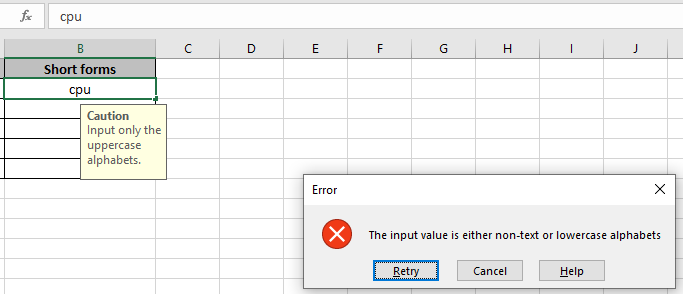In this article, we will learn how to validate only uppercase text entries in Excel 2016.

Problem:

Here we have a task where only uppercase text entries are allowed. So we need to check two conditions at the same time.

• The value must be text.
• The text must be upper case.

How to solve the Problem:

Validation of uppercase text entries is done with the use of  Excel functions. The following steps will be a guide, how to validate text entries.

The use of functions will help us get through the situation.

We will construct a formula out of it. First the text would be checked that it is text or not. Then the text will have to match with the uppercase of the same text. Last but not the least AND operator will check if only the above two conditions matches.

Generic formula:

= AND ( EXACT ( cell , UPPER ( cell ) ) , ISTEXT ( cell ) )

Explanation:

1. ISTEXT function checks the value for the text.
2. UPPER function converts the value to uppercase.
3. EXACT function matches the value with its own uppercase value.
4. AND operator returns TRUE if and only if the both conditions returns TRUE.

Let's get this through with this via using the formula using .

Here we have a list of Full forms and we need to fill the short form list.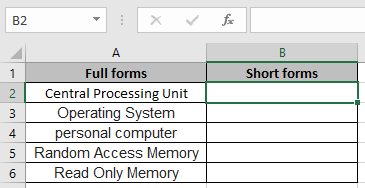But we need to lock these input cells with some rules. Now we learn how to do it.

We will use the data validation attribute of excel. Follow the steps to do the same:

1. Select the cells where data validation need to apply.
2. Click Data > Data Validation option from the menu.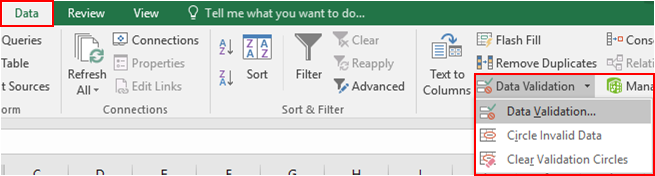3. The Data validation dailog box appears in front.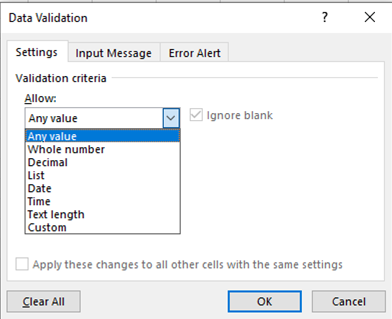4. In the Settings option, select the Custom option from the Allow list.

5. The formula box will appear under the same option.
6. We will construct a formula out of it. First the text would be checked that it is text or not. Then the text will have to match with the uppercase of the same text. Last but not the least AND operator will check if only the above two conditions matches.
7. Use the formula in the formula box.

= AND ( EXACT ( B2 , UPPER ( B2 ) ) , ISTEXT ( B2 ) )

Explanation:

1. ISTEXT function checks the value in  for the text.
2. UPPER function converts the value to uppercase.
3. EXACT function matches the value with its own uppercase value.
4. AND operator returns TRUE if and only if the both conditions returns TRUE.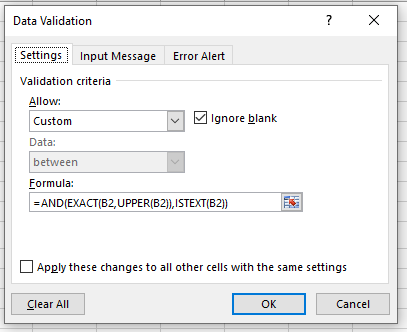7. Fill in the details as shown above. Click OK

Now when I enter the value as shown below. A default error generates.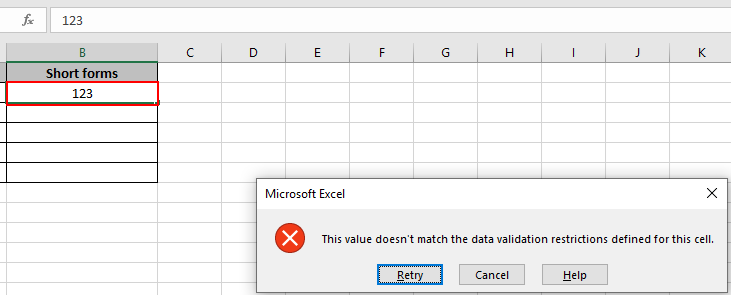As you can see an error is generated "This value doesn't match the data validation restrictions defined for this cell".

But now the problem is how the user knows what restrictions are there and what type of value the cell can accept.

So for this, we will customize some changes as shown below.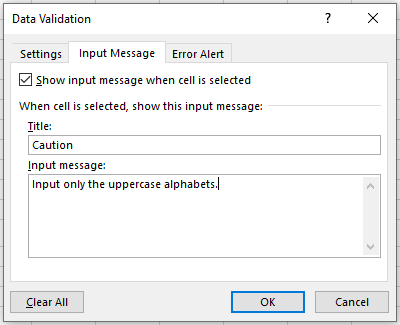In the Input Message option, type the details under Title & Input Message box as shown above.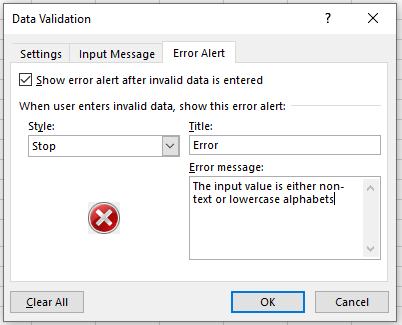In Error alert option, type the details under Title & Error Message box as shown above.

Click Ok.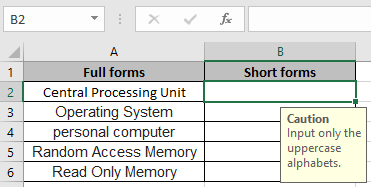A message is displayed when the cell is selected and an error message is displayed as shown below.Now fill in the right answers to complete the table.

To copy the data validation to the adjacent cells use the drag down or drag right option in excel.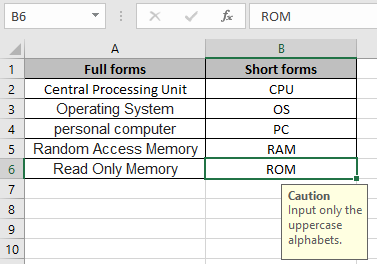Now our table is complete. Now it's user friendly and informative as well.

Hope you understand how to Validate only uppercase data validation in Excel. Explore more articles on Accounting here.  Please write your queries in the comment box below. We will help you.

Related Articles:

Data Validation in Excel : restricts user to input value manually in Excel.

Way to use Vlookup function in Data Validation : allow values from the vlookup table in Excel.

Restricted Dates using Data Validation : allow dates in the cell which lays within Excel date format.

How to give the error messages in Data Validation : customize your data validation cell with error messages.

Create Drop Down Lists in Excel using Data Validation : restricts user to input data manually.

Popular Articles

50 Excel Shortcut to Increase Your Productivity

Edit a dropdown list

Absolute reference in Excel

If with conditional formatting

If with wildcards

Vlookup by date

Convert Inches To Feet and Inches in Excel 2016

Join first and last name in excel

Count cells which match either A or B

Terms and Conditions of use

The applications/code on this site are distributed as is and without warranties or liability. In no event shall the owner of the copyrights, or the authors of the applications/code be liable for any loss of profit, any problems or any damage resulting from the use or evaluation of the applications/code.